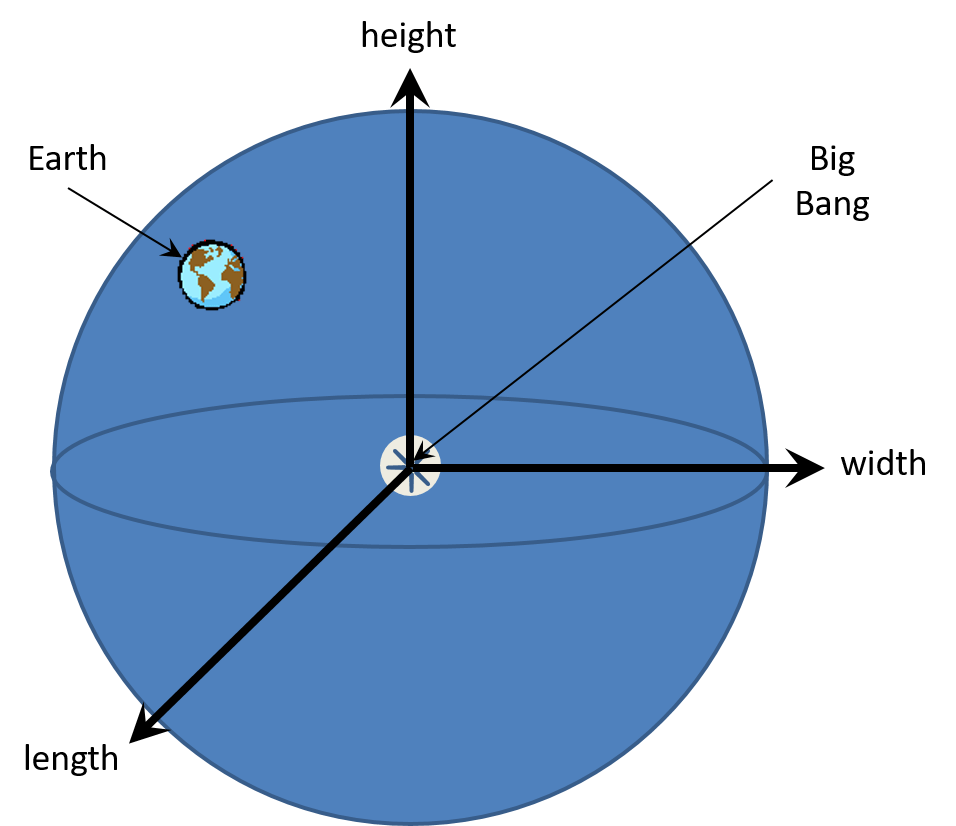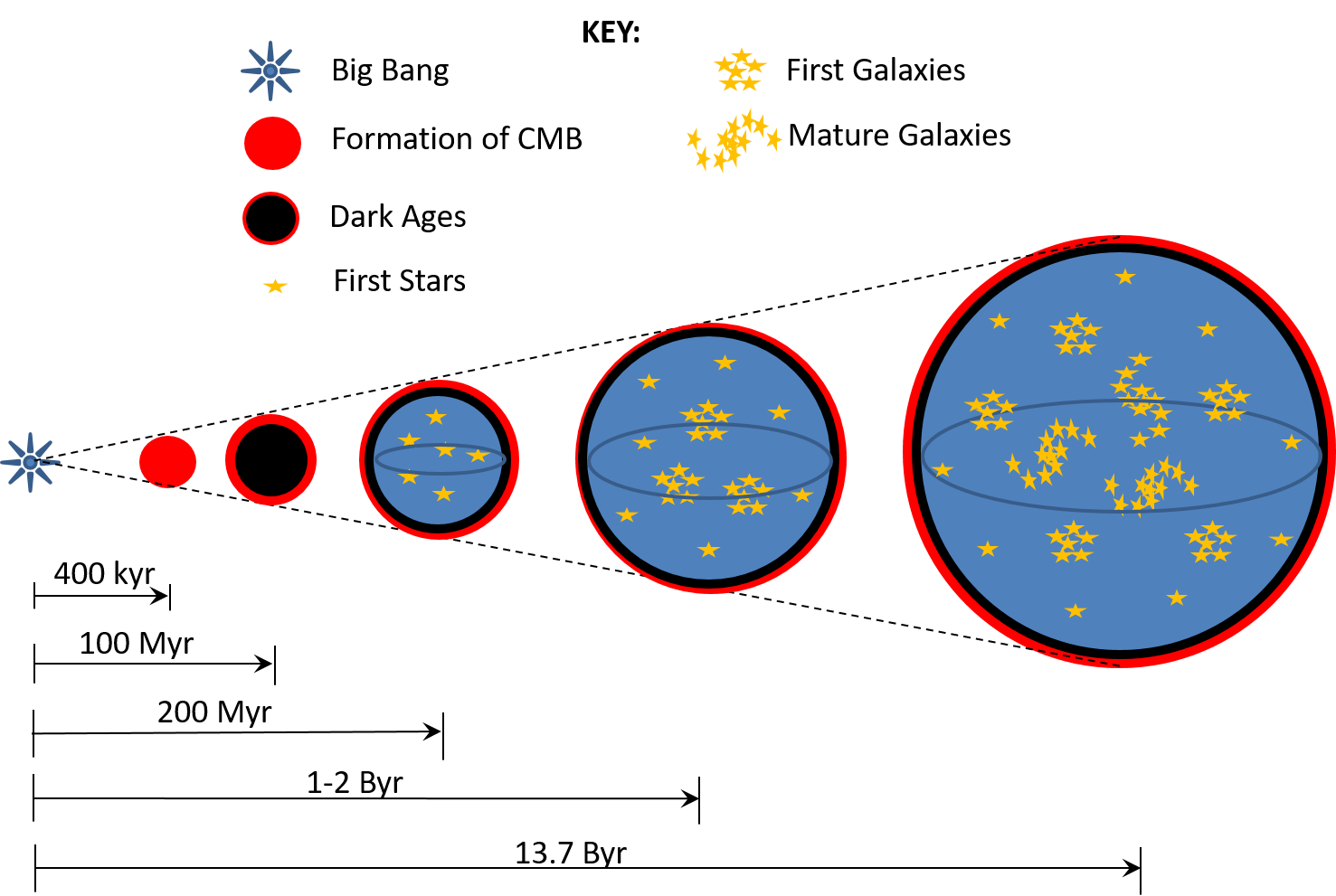# Spacetime

Spacetime is a four-dimensional system that is composed of three spatial dimensions and a temporal time dimension. Einstein used the concept of spacetime in his General Theory of Relativity to explain gravity. Einstein borrowed the concept of spacetime from Hermann Minkowski, one of Einstein’s teachers. Under Minkowski’s spacetime model, there is no requirement for the three spatial dimensions to be orthogonal. Einstein liked the idea that spatial dimensions may be non-Euclidean in nature and that the temporal time dimension could use the Lorentz Factor to represent gravitational time dilation. We have all seen pictures of how massive objects warp spacetime as illustrated below:Minkowski’s spacetime, in general, is not limited to four dimensions. It can consist of any number of dimensions. The specific assumptions made by Einstein’s in his use of the spacetime model in his General Theory of Relativity are the following:

·         The spatial dimensions are non-Euclidean,
·         There are only three spatial dimensions,
·         The temporal time dimension is based on gravitational time dilation.

We think of spatial dimensions as things that have length, width, and height. These three dimensions are as obvious to us as the geocentric universe of Ptolemy where the Sun, Moon and stars all orbit around the Earth. We have no sensation of the Earth moving, and we can see the parade of celestial objects move from east to west in the sky above us. However, we now know our senses can be deceiving. Only the Moon orbits around the Earth. The Earth orbits around the Sun. The Sun orbits around the Milky Way galaxy, and the Milky Way galaxy orbits around the center of mass of our Local Group of galaxies. Without scientific inquiry, we would still be thinking the universe is geocentric.

One question to be asked is whether our universe is non-Euclidean. Einstein argued that, while our universe may look Euclidean in our immediate neighborhood, the universe as a whole is not Euclidean. Einstein’s conclusion of a non-Euclidean universe is based on the argument that massive objects bend the three spatial dimensions to create gravity wells. Objects of lesser mass follow a geodesic path through these gravity wells. A geodesic path is like a straight line in Euclidean geometry. Just as a straight line is the shortest path through Euclidean space, a geodesic is the shortest path through a non-Euclidean space. It is these gravity wells and the speed of the smaller object that determines whether the inertia of a smaller object follows a hyperbolic or parabolic path around the larger object, or even goes into orbit around the larger object.

The issue of what is gravity has perplexed scientists throughout the ages. Under Newtonian gravity, there was a general belief that objects of mass pulled on each other. Particle physicists are searching for an elusive graviton particle that supposedly is exchanged between objects of mass to pull them together. However, the distance between objects in outer space is so astronomical that it is unlikely that gravity is a pulling force.

Einstein theorized that gravity is the bending of spacetime caused by the mass of an object moving through space. Gravity, according to Einstein is nothing more than an object following the shortest path (i.e. geodesic) under its own inertia through a warped spacetime. John Wheeler, an American theoretical physicist, described Einstein’s view of gravity as “spacetime tells matter how to move. Matter tells spacetime how to curve.”

However, one never sees this warping of space. When one is at a soccer game and a player kicks a ball high in the air down the field, there is no visual warping causing the ball to follow a geodesic path. In fact. Our space probes are telling us our universe is flat meaning it follows Euclidean geometry. Both the United State’s Wilkinson Microwave Anisotropy Probe (WMAP) and the European Space Agency’s Planck probe have confirmed this flatness of the universe.

Since we now have scientific confirmation that our spatial dimensions are Euclidean, let us examine the claim there are only three spatial dimensions. Of interest to us is the location of the Big Bang. There is no one point in the night sky where we can point and say that is the direction where the Big Bang occurred. But, if the universe is made up of three Euclidean dimensions, there must be a point in the night sky where the Big Bang occurred.

Consider the following diagram of three spatial dimensions where each axis is orthogonal (i.e. 90°) to each other (i.e. a Euclidean space):If there was a Big Bang, then we should be able to place the origin of our three-dimensional Euclidean space at the point of the Big Bang. The universe continues to expand in all three directions. Our Earth today exists somewhere in this expanding space. Mathematically, the Big Bang must exist at a single point in the sky. We should see a progression of younger galaxies, and then a progression of older galaxies as we peer deeper in that one direction. However, deep space studies using the Hubble Space Telescope reveals that we see a progression of only younger galaxies in every direction we look. The scientific conclusion here is that the Big Bang occurred at the farthest distances in all directions.

The only way this can happen mathematically is for our universe to have moved away from the point of the Big Bang as illustrated below:What we see in this illustration is a progression of our three-dimension universe moving away from the location of the Big Bang. The fact the universe can move away from the Big Bang means there must be a fourth spatial dimension, called the Spatial-Time Dimension, through which our universe is moving. This dimension must exist in the Multiverse – a higher order area of space that may contain multiple universes. The scientific evidence of the Hubble Space Telescope’s deep space studies leads us to the conclusion that our Multiverse must be at least a four-dimensional Euclidean space.

Finally, let us address the issue of the temporal time dimension being based on gravitational time dilation. As we saw in the assumption regarding the Speed of Light, it is the idea of a constant speed of light that leads to a conclusion that time dilation exists. The movement of photons at the constant speed c through gravitational forces leads to the concept of gravitational time dilation. By recognizing photons have a mass equivalency, they are affected by gravitational fields. We know for a fact that the path of photons bends as they pass a massive object such as the Sun or a galaxy. If photons respond to gravitational fields, then they must also accelerate and decelerate due to gravitational fields. The conclusion we reached in the assumption of the Speed of Light article is that light has a non-constant velocity. As a result, there is no such thing as gravitational time dilation.

The Romeo Effect series of books accepts the Minkowski spacetime model, but assumes the following is true:

·         The spatial dimensions are Euclidean,
·         There are at least four spatial dimensions,
·         The temporal time dimension is linear.

Logically, this type of spacetime model seems more intuitive than the spacetime model used by Einstein. The only issue with this new model is we still do not understand the nature of gravity. Book 4 in the Romeo Effect series of books will propose a new explanation of gravity.